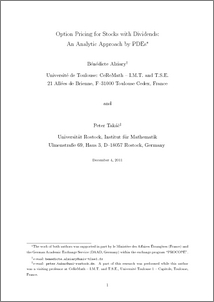# Option Pricing for Stocks with Dividends: An Analytic Approach by PDEs

Alziary Chassat, Bénédicte and Takac, Peter (2012) Option Pricing for Stocks with Dividends: An Analytic Approach by PDEs. Monografıas de la Real Academia de Ciencias de Zaragoza (n° 38). pp. 125-136.Preview
PDF

## Abstract

We study the Black-Scholes equations for pricing options on stocks by splitting it into two simpler PDEs that can be solved by analytically simpler and numerically faster methods than the original Black-Scholes PDE. We first use a deflator process to arrive at a numeraire S(interest-neutral stock price) computed from the first equation and then obtain a simple Black-Scholes equation for the interest-neutral call option price P with no explicit dependence on the (instantaneous short) interest rate r. We also formulate two theorems on the solvability of these PDEs.

Item Type: Article English 2012 Yes G- MATHEMATIQUES Institut de mathématiques de Toulouse, TSE-R (Toulouse) UT1 23 Jan 2013 12:20 26 Feb 2020 15:08 http://publications.ut-capitole.fr/id/eprint/8631View Item